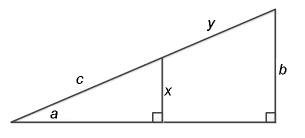Name:    Math 10W Practice Unit Test #5

Multiple Choice
Identify the choice that best completes the statement or answers the question.

1.

A ladder is 5 m long. If it is leaning against a wall, with the bottom of the ladder 1.5 m away from the base of the wall, how high up the wall will it reach?
 a. 2.57 m c. 3.67 m b. 5.22 m d. 4.77 m

2.

What is the sine of 47°?
 a. 0.682 c. 0.731 b. 1.072 d. 0.765

3.

A right triangle has a hypotenuse of 16 cm. If one of the angles is 27°, what is the length of the opposite side?
 a. 8.15 cm c. 7.26 cm b. 8.47 cm d. 14.26 cm

4.

A right triangle has an angle of 68° and the opposite side is 31 cm. What is the length of the hypotenuse?
 a. 12.52 cm c. 82.75 cm b. 33.43 cm d. 44.73 cm

5.

What is the cosine of 59°?
 a. 0.857 c. 1.664 b. 0.515 d. 0.891

6.

A right triangle has an angle of 59° and the adjacent side is 30 cm. What is the hypotenuse?
 a. 35.00 cm c. 46.30 cm b. 18.03 cm d. 58.25 cm

7.

A right triangle has a leg of 3.6 cm and the adjacent angle is 35.4°. What is the length of the hypotenuse?
 a. 6.21 cm c. 5.07 cm b. 17.51 cm d. 4.42 cm

8.

A right triangle has an angle of 15°. If the opposite side is 67 cm long, what is the length of the adjacent side?
 a. 250.0 cm c. 253.7 cm b. 258.9 cm d. 254.9 cm

9.

A right triangle has an angle of 76°. If the adjacent side is 19.0 cm long, what is the length of the opposite side?
 a. 19.0 cm c. 76.2 cm b. 74.5 cm d. 78.5 cm

10.

A right triangle has one angle of 34°. If the side opposite the given angle measures 15.3 cm, what is the length of its adjacent side?
 a. 22.68 cm c. 18.46 cm b. 38.56 cm d. 27.36 cm

1.

A right triangle has a hypotenuse of 24 m. If one of the angles is 54°, what is the length of the opposite side?

2.

A road worker measures the incline of a ramp that rises 11.5 m to be 25.1°. What is the length of the ramp?

3.

The diagram below has the following dimensions:
a = 27°
b = 35 cm
c = 36 cm

What is the value of y?4.

A worker uses a board to create a temporary ramp for his wheelbarrow. If the board covers a horizontal distance of 107 cm and has an angle of elevation of 21.9°, how long is the board, in metres?

5.

Gerald wants to use his kite to see if he can successfully drop a water balloon on an unsuspecting target. If his kite is flying at an angle of 70° from the ground and his target is 12 m away, how much string does Gerald need to let out on his kite?